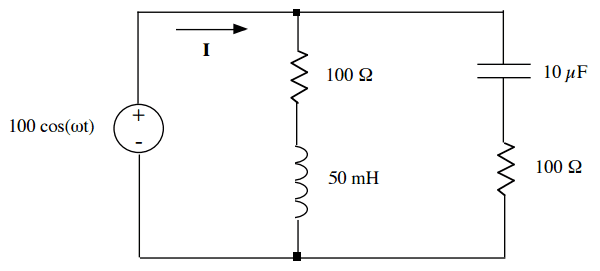# Find Frequency for Zero Reactance in AC Circuit

• Engineering
• trynalearn

#### trynalearnThe question is:

The voltage source in the above circuit is a sinusoidal AC source with constant amplitude and constant phase shift but an adjustable frequency.
Calculate the frequency ω at which the phasor current I will have zero phase shift relative to the
voltage source. In other words, the equivalent impedance across the voltage source behaves like a
pure resistance with zero reactance at the required frequency.
(HINT: Given two complex numbers such that A + jB = C + jD, then A = C and B = D by inspection,
i.e. the real portion must equal the real portion and the imaginary portion must equal the
imaginary portion.)

Attempt:

Since it stated that the reactance is zero, that means Z = R. So Req = (1/100+1/100)^-1 = 50. I converted the v(t) into V (phasors) which become 100. Then I = V/R, so I get 100/50 = 2. I don't know what to do next. Am I even doing it correctly?

Suggestion: when will the two reactances be equal and opposite? (giving zero sum)

Last edited by a moderator:
Hello trying, welcome to PF!

You are doing fine. What about the hint in the exercise ? You have ## {1\over Z_{\rm total} } = {1\over Z_1 } + {1\over Z_2 } ## with 1 for branch 1 and 2 for branch 2, and all ## Z ## complex, but the imaginary part of ## {Z_{\rm total} }## equal to 0 ...

During resonance?

In other words, the equivalent impedance across the voltage source behaves like a
pure resistance with zero reactance at the required frequency
At the frequency the exercise is asking for Z = R, a real quantity.

At the frequency the exercise is asking for Z = R, a real quantity.
building off what BvU said, why don't you start by getting an algebraic expression for Z